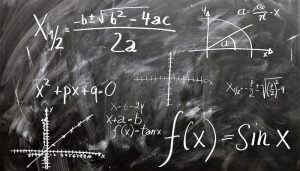Mathematics

# Algebraic numbers

Within the set of real numbers we can find different types of classes of numbers such as rational and irrational numbers, which in turn can be subdivided into others, among which we can mention the algebraic numbers, that are the set of numbers that appear as a result of an algebraic equation. They cannot be expressed in fractions and can represent the real root of a polynomial of degree "n". Using these numbers we can observe the processes of resolution of algebraic operations of polynomials and in formulas of quantum physics and factual sciences.Related topics

## What are algebraic numbers?

Algebraic numbers are part of the set of irrational or rational numbers that result from the solution of an algebraic equation represented in real or complex numbers. They are numbers with non-recurring decimals such as non-exact roots. Irrational numbers that are not algebraic are called transcendent.

## Which are the algebraic numbers?

Algebraic numbers are any real root of a polynomial of degree “n” with integer coefficients. These can be real numbers and complex numbers.

Imaginary numbers are algebraic just like real numbers.

## What are they for?

They are used for the resolution of algebraic operations such as polynomials of grade n.

It is important to remember that the algebraic equation is always a polynomial with real or complex coefficients equal to zero.

Algebraic numbers are widely used in sciences such as statistics, mathematics, physics, chemistry, astronomy and others.

## Characteristics

Among the characteristics of algebraic numbers we can mention the following:

• They are part of the set of irrational numbers and rational numbers.
• They can be imaginary numbers.
• They are all real and complex.
• The set of algebraic numbers is numerable.
• Rational numbers are algebraic but irrational numbers may not be.
• They are definable.
• They are countable.
• They are grade n if it is the root of an algebraic equation of grade n, but it is not the root of an algebraic equation of grade n-1.

## History

The history of algebraic numbers begins with the Swiss mathematician and philosopher Leonhard Euler, who in 1748 proposes the division between two types of irrational numbers, transcendent and algebraic.

Some years later, in 1844, the French mathematician Joseph Liouville developed the first criterion to determine that a number is algebraic and transcendent. Then, between 1874 and 1895, the theory of algebraic numbers was further expanded thanks to the studies carried out by the German mathematician Richard Derekind and the Russian Yegor Zolotariov.

According to many mathematicians, the basis of this theory of algebraic integers was created by the German mathematician Karl Gauss and then developed and deepened by the mathematician Ernst Kummer.

## How algebraic numbers are represented?

Unlike other complex numbers, algebraic numbers do not have a letter to represent them. In spite of not having a letter that represents this set of numbers, there is an element that represents then, and it is the polynomial. These polynomials can be of different degrees equaled to zero.

## Operations

Algebraic numbers, being the result of equations, can be used in different types of operations such as addition, subtraction, multiplication or division in the polynomials of degree n where they are present.

It is important to mention that the addition, difference, product or quotient of two algebraic numbers will always result in another algebraic number.

When a non-zero number αα is the solution to an equation anxn+⋯+a1x+a0=0anxn+⋯+a1x+a0=0

Then the numbers -α-α and 1/α1/α are roots of the equations (-1) nanxn+⋯-a1x+a0=0(-1) nanxn+⋯-a1x+a0=0 et a0xn+⋯+an-1x+an=0a0xn+⋯+an-1x+an=0

As a consequence of this, the opposite of an algebraic number other than zero is an algebraic number. It can be shown that the sum and the product of two algebraic numbers are also algebraic numbers, but that this result is much more complex and is usually obtained through the theory of body extensions.

## Examples of algebraic numbers

Here are some examples of algebraic numbers. These are:

• √2 = x2-2= 0
• X3– 5 = 0
• 8x3 – 3 = 0
• 6x2 +7x- √334=0
• 9x2+ √584=0
• √32 = x2+x-3= 0
• X3 -17x = 0
• 95x3 +(3x- 3) = 0
• √934x + 6x2 +7x =0
• (77x2+x)-951 =0
Written by Gabriela Briceño V.